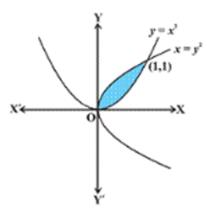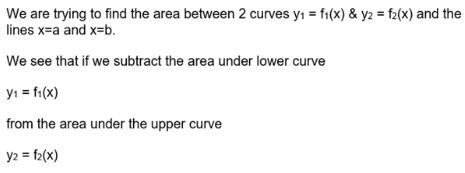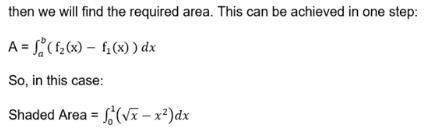# Test: Area Between Two Curves

## 10 Questions MCQ Test Mathematics (Maths) Class 12 | Test: Area Between Two Curves

Description
This mock test of Test: Area Between Two Curves for JEE helps you for every JEE entrance exam. This contains 10 Multiple Choice Questions for JEE Test: Area Between Two Curves (mcq) to study with solutions a complete question bank. The solved questions answers in this Test: Area Between Two Curves quiz give you a good mix of easy questions and tough questions. JEE students definitely take this Test: Area Between Two Curves exercise for a better result in the exam. You can find other Test: Area Between Two Curves extra questions, long questions & short questions for JEE on EduRev as well by searching above.
QUESTION: 1

### The area between the curves y = x2 and y = x3 is:​

Solution:

y = x2 and y = x3
To find point of intersections :
x2 = x3
x2(x-1) = 0
So, x = 0,1
POI are (0,0) & (1,1)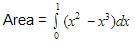= [x3/3 – x4/4]x=1   - [x3/3 – x4/4]x=0
= (1/3 – 1/4) – (0)
= 1/12 sq units

QUESTION: 2

Solution:
QUESTION: 3

### The shaded area is equal to: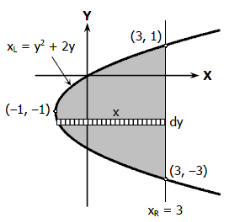​

Solution: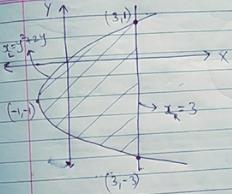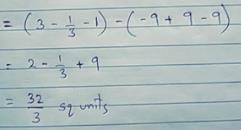QUESTION: 4

The curves x2 + y2=16 and y2 = 6x intersects at​

Solution:

x2 + y2=16 and y2 = 6x
So, to figure out the P.O.I
x2 + 6x = 16
x2 + 6x – 16 = 0
(x+8) (x-2) = 0
x = -8, 2
For x = -8
y = (6*(-8))1/2
= (-48)1/2
No real value for y
For x = 2
y = (6*2)1/2
= (12)1/2
= 2*(3)1/2
So, P.O.I is (2 , 2*(3)1/2 )

QUESTION: 5

Find the area of region bounded by curve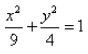Solution: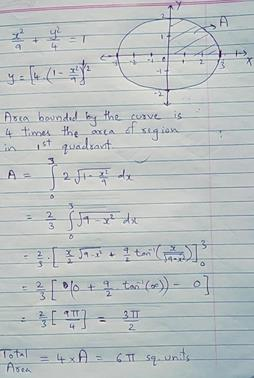QUESTION: 6

The area of the region bounded by y = x2 – 2x and y = 4 – x2 is.​

Solution:

To find area between y = x2 – 2x and y = 4 – x2,
We need to find POI.
x2 – 2x = 4 – x2
2x2 - 2x – 4 = 0
x2 – x – 2 = 0
(x-2)(x+1) = 0
x = -1, 2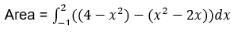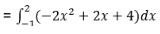= [(-2x3)/3 + x2 + 4x]x=2 - [(-2x3)/3 + x2 + 4x]x= -1
= -16/3 + 4 + 8 – (2/3 + 1 - 4)
= 9 sq units

QUESTION: 7

The shaded area enclosed between the parabolas with equations y = 1 + 10x – 2x2and y = 1 + 5x – x2 is equal to:

Solution: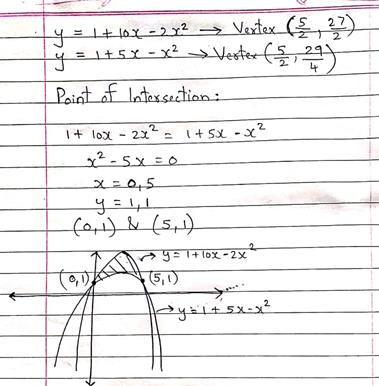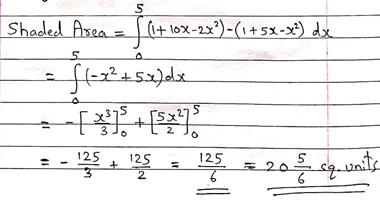QUESTION: 8

The area of the smaller region lying above the x-axis and included between the circle x2 + y2 = 2x and the parabola y2 = x.

Solution: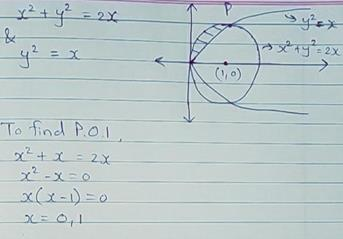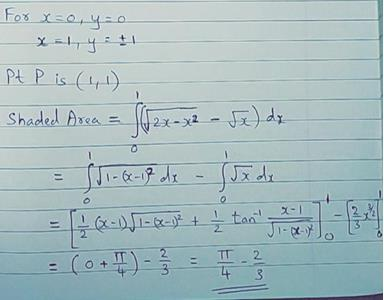= π/4 – 2/3

QUESTION: 9

The area bounded by the curves f(x) = x2 + 1 and g(x) = x – 1 on the interval [1,3] is:​

Solution: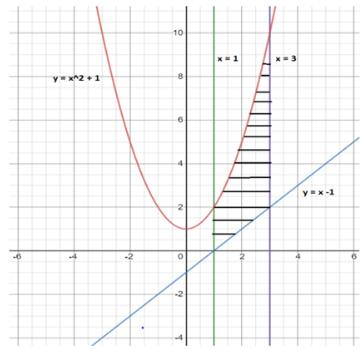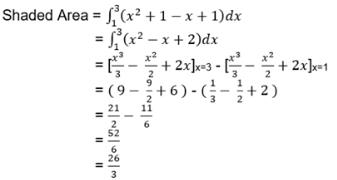QUESTION: 10

The area shaded in the given figure can be calculated by which of the following definite integral?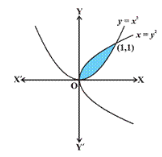Solution: